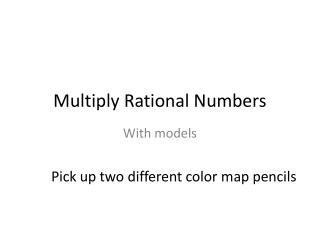DownloadDownload PresentationMultiply Rational Numbers

# Multiply Rational Numbers

Download Presentation## Multiply Rational Numbers

- - - - - - - - - - - - - - - - - - - - - - - - - - - E N D - - - - - - - - - - - - - - - - - - - - - - - - - - -
##### Presentation Transcript

1. Multiply Rational Numbers With models Pick up two different color map pencils

2. A Decimal is a special part of a whole. A decimal is always divided into powers of 10. Name 1 whole 1 hundredths 1 tenth Decimal 1.0 0.01 0.1 1/100 1/10 1/1 Fraction

3. 0.7 0.8 0.56 You can use decimal grids to model multiplication of decimals. The area where the shading overlaps shows the product of the two decimals. Color Vertically Color Horizontally Overlap  = 56/100 8/10 7/10

4. = 0.4 0.2 0.08 2/10 1/5 8/100 2/25 4/10 2/5

5. 4/5 = X 3/8 12/40 = 3/10

6. Notes start here

7. Date __________________ Multiply Rational Numbers With models

8. A Decimal is a special part of a _____. A decimal is always divided into ______ __ __. Name Decimal Fraction

9. You can use decimal grids to model multiplication of decimals. The area where the shading overlaps shows the product of the two decimals. Color Vertically Color Horizontally Overlap  =

10. =